# NCRT Miscellaneous Exercise

Question 1: Let f: R → R be defined as f(x) = 10x + 7. Find the function g: R → R such that

gof = fog = IR.

It is given that f: R → R is defined as f(x) = 10x + 7.

For one – one

Let f(x) = f(y), where x, y ∈ R.

⇒ 10x + 7 = 10y + 7

⇒ x = y

∴ f is a one – one function.

For onto

For y ∈ R, let y = 10x + 7.

⇒ x = (y-7) / 10 ∈ R

Therefore, for any y ∈ R, there exists x = (y-7) / 10 ∈ R such that

f(x) = f ((y−7) / 10)) = 10 ((y−7)/10)) + 7 = y − 7 + 7 = y

∴ f is onto.

Therefore, f is one – one and onto.

Thus, f is an invertible function.

Let us define g: R → R as g(y) =(y−710)

Now, we have

gof(x) = g(f(x)) = g(10x + 7) = ((10x + 7) -7) / 10)))=(10x / 10) = x

and

fog(y) = f(g(y)) = f((y - 7)/10)) = 10((y - 7)/10))+7 = y-7+7 = y

∴ gof = IðÂ‘… and fog = IR.

Hence, the required function g: R → R is defined as g(y) =(y−7) /10.

Question 2:

Let f: W → W be defined as f(n) = n − 1, if is odd and f(n) = n + 1, if n is even.

Show that f is invertible. Find the inverse of f. Here, W is the set of all whole

numbers.

It is given that:

f: W → W is defined as f(n) =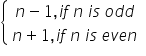For one – one

Let f(n) = f(m).

It can be observed that if n is odd and m is even, then we will have n − 1 = m + 1.

⇒ n − m = 2

However, this is impossible.

Similarly, the possibility of n being even and m being odd can also be ignored

under a similar argument.

∴ Both n and m must be either odd or even. Now, if both n and m are odd,

Then, we have

f(n) = f(m)

⇒ n − 1 = m – 1

⇒ n = m

Again, if both n and m are even,

Then, we have

f(n) = f(m)

⇒ n + 1 = m + 1

⇒ n = m

∴ f is one – one.

For onto

It is clear that any odd number 2r + 1 in co-domain N is the image of 2r in domain

N and any even number 2r in co-domain N is the image of 2r + 1 in domain N.

∴ f is onto.

Hence, f is an invertible function.

Let us define g: W → W as g(m) =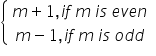Now, when n is odd

gof(n) = g(f(n)) = g(n − 1) = n − 1 + 1 = n and

When n is even

gof(n) = g(f(n)) = g(n + 1) = n + 1 - 1 = n

Similarly,

When m is odd

fog(m) = f(g(m)) = f(m − 1) = m − 1 + 1 = m and

When m is even

fog(m) = f(g(m)) = f(m + 1) = m + 1 - 1 = m

∴ gof = IW and fog = IW

Thus, f is invertible and the inverse of f is given by f-1= g, which is the same as

f.  Hence, the inverse of f is f itself.

Question 3:

If f: R → R is defined by f(x) = x2− 3x + 2, find f(f(x)).

It is given that f: R → R is defined as f(x) = x2− 3x + 2.

f(f(g)) = f(x2-3x + 2)

=(x2-3x + 2)2 - 3(x2-3x + 2) +2

=(x4-9x2 + 4 - 6x3-12x + 4x2) + (-3x2-9x - 6)+2

=x4-6x3 + 10x2 - 3x

Question 4:

Show that function f: R → {x ∈ R: −1 < x < 1} defined by f(x) =(x /(1+|x|)), x ∈ R is

one – one and onto function.

It is given that f: R → {x ∈ R: −1 < x < 1} is defined as f(x)  =(x/(1+|x|)), x ∈ R.

For one – one

Suppose f(x) = f(y), where x, y ∈ R.

⇒(x/(1 + |x|)) =(y/(1 + |y|))

It can be observed that if x is positive and y is negative,

Then, we have

(x/(1 + x)) =(y/(1 - y))⇒ 2xy = x – y

Since, x is positive and y is negative

x > y ⇒ x − y > 0

But, 2xy is negative.

Then 2xy ≠ x – y

Thus, the case of x being positive and y being negative can be ruled out.

Under a similar argument, x being negative and y being positive can also be ruled

out.

∴  x and y have to be either positive or negative.

When x and y are both positive, we have

⇒ f(x) = f(y) ⇒(x/(1 + x))=(y/(1 + y)) ⇒ x + xy = y + xy ⇒ x = y

When x and y are both negative, we have

⇒ f(x) = f(y) ⇒(x/(1 - x))=(y/(1 - y)) ⇒ x - xy = y - xy ⇒ x = y

∴ f is one – one.

For onto

Now, let y ∈ R such that −1 < y < 1.

If y is negative, then, there exists x =(y / (1+y)) ∈ R such thatIf y is positive, then, there exists x =(y(1−y)) ∈ R such that∴ f is onto.

Hence, f is one – one and onto.

Question 5: Show that the function f: R → R given by f(x) = x3 is injective.

f: R → R is given as f(x) = x3.

For one – one

Suppose f(x) = f(y), where x, y ∈ R

⇒ x3= y3 ………………………………… (1)

Now, we need to show that x = y.

Suppose x ≠ y, their cubes will also not be equal.

⇒ x3 ≠ y3

However, this will be a contradiction to (1).

∴ x = y

Hence, f is injective.

Question 6:

Give examples of two functions f: N → Z and g: Z → Z such that g o f is injective

but g is not injective.

(Hint: Consider f(x) = x and g (x) = |x|)

Define f: N → Z as f(x) = x and g: Z → Z as g (x) = |x|.

We first show that g is not injective.

It can be observed that

g (−1) =|−1| = 1

g (1) = |1| = 1

∴ g (−1) = g (1), but −1 ≠ 1.

∴ g is not injective.

Now, g of: N → Z is defined as go(x) = g(f(x)) = g(x) = |x|.

Let x, y ∈ N such that g of(x) = g of(y).

⇒ |x| = |y|

Since x and y ∈ N, both are positive.

∴ |x| = |y| ⇒ x = y

Hence, gof is injective

Question 7: Given examples of two functions f: N → N and ðÂ‘”: N → N such that ðÂ‘” of is onto but f is not onto.

(Hint: Consider f(x) = x + 1 and (ðÂ‘¥) =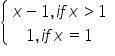Define f: N → N by f(x) = x + 1

and g: N → N by g(x) =We first show that g is not onto.

For this, consider element 1 in co-domain N. It is clear that this element is not an image of any of the elements in domain N.

∴ f is not onto.

Now, gof: N → N is defined by

go(x) = g(f(x)) = g(x + 1) = x + 1 − 1 = x               [x ∈ N ⇒ x + 1 > 1]

Then, it is clear that for y ∈ N, there exists x = y ∈ N such that gof(x) = y. Hence, ðÂ‘”of is onto.

Question 8: Given a non-empty set X, consider P(X) which is the set of all subsets of X. Define the relation R in P(X) as follows:

For subsets A, B in P(X), ARB if and only if A ⊂ B. Is R an equivalence relation on P(X)? Justify you answer.

Since every set is a subset of itself, ARA for all A ∈ P(X).

∴ R is reflexive. Let ARB ⇒ A ⊂ B. This cannot be implied to B ⊂ A. For instance, if A = {1, 2} and B = {1, 2, 3}, then it cannot be implied that B is related to A.

∴ R is not symmetric. Further, if ARB and BRC, then A ⊂ B and B ⊂ C.

⇒ A ⊂ C ⇒ ARC

∴ R is transitive.

Hence, R is not an equivalence relation as it is not symmetric.

Question 9: Given a non-empty set X, consider the binary operation *: P(X) × P(X) → P(X) given by A * B = A ∩ B ∀ A, B in P(X) is the power set of X. Show that X is the identity element for this operation and X is the only invertible element in P(X) with respect to the operation*.

It is given the binary operation *:

P(X) × P(X) → P(X) given by A * B = A ∩ B ∀ A, B in P(X)

We know that A ∩ X = A = X ∩ A for all A ∈ P(X)

⇒ A * X = A = X * A for all A ∈ P(X)

Thus, X is the identity element for the given binary operation *.

Now, an element A ∈ P(X) is invertible if there exists B ∈ P(X) such that

A * B = X = B * A              [As X is the identity element]

or

A ∩ B = X = B ∩ A

This case is possible only when A = X = B.

Thus, X is the only invertible element in P(X) with respect to the given operation*. Hence, the given result is proved.

Question 10: Find the number of all onto functions from the set {1, 2, 3, … , n) to itself.

Onto functions from the set {1, 2, 3, … , n} to itself is simply a permutation on n symbols 1, 2, …, n.

Thus, the total number of onto maps from {1, 2, … , n} to itself is the same as the total number of permutations on n symbols 1, 2, …, n, which is n.

Question 11: Let S = {a, b, c} and T = {1, 2, 3}. Find F−1 of the following functions F from S to T, if it exists. (i) F = {(a, 3), (b, 2), (c, 1)}             (ii) F = {(a, 2), (b, 1), (c, 1)}

S = {a, b, c},        T = {1, 2, 3}

(i) F: S → T is defined as F = {(a, 3), (b, 2), (c, 1)}

⇒ F (a) = 3, F (b) = 2, F(c) = 1

Therefore, F−1 : T → S is given by F−1 = {(3, a), (2, b), (1, c)}.

(ii) F: S → T is defined as F = {(a, 2), (b, 1), (c, 1)}

Since F (b) = F (c) = 1, F is not one – one.

Hence, F is not invertible i.e., F−1 does not exist.

Question 12: Consider the binary operations *: R × R → R and o: R × R → R defined as a ∗ b = |a − bÂ| and a o b = a, ∀ a, b ∈ R. Show that * is commutative but not associative, o is associative but not commutative. Further, show that ∀ a, b, c ∈ R, a*(b o c) = (a * b) o (a * c). [If it is so, we say that the operation * distributes over the operation o]. Does o distribute over *? Justify your answer.

It is given that *: R × R → and o: R × R → R is defined as a ∗ bÂ = |a − bÂ| and a o b = a, ∀ a, b ∈ R For a, b ∈ R, we have a ∗ b = |a − bÂ| and bÂ ∗ a = |bÂ − a| = |−(a − b)| = |a − bÂ| ∴ a * b = b * a

Hence, the operation * is commutative.

It can be observed that

(1 ∗ 2) ∗ 3 = (|1 − 2|) ∗ 3 = 1 ∗ 3 = |1 − 3| = 2

and

1 ∗ (2 ∗ 3) = 1 ∗ (|2 − 3|) = 1 ∗ 1 = |1 − 1| = 0

∴ (1 ∗ 2) ∗ 3 ≠ 1 ∗ (2 ∗ 3) where 1, 2, 3 ∈ R.

Hence, the operation * is not associative.

Now, consider the operation o

It can be observed that 1 o 2 = 1 and 2 o 1 = 2.

∴ 1 o 2 ≠ 2 o 1 where 1, 2 ∈ R.

Hence, the operation o is not commutative.

Let a, b, c ∈ R. Then, we have

(a o b) o c = a o c = a

and

a o (b o c) = a o b = a

∴ a o b) o c = a o (b o c), where a, b, c ∈ R

Hence, the operation o is associative.

Now, let a, b, c ∈ R, then we have

a * (b o c) = a * b =|a − bÂ|

(a * b) o (a * c) = (|a − bÂ|)o(|a − b|) = |a − bÂ|

Hence, a * (b o c) = (a * b) o (a * c).

Now,

1 o (2 ∗ 3) = 1(|2 − 3|) = 1o1 = 1

(1 o 2) * (1 o 3) = 1 * 1 = |1 − 1| = 0

∴ 1 o (2 * 3) ≠ (1 o 2) * (1 o 3) where 1, 2, 3 ∈ R

Hence, the operation o does not distribute over *.

Question 13: Given a non -empty set X, let *: P(X) × P(X) → P(X) be defined as A * B = (A − B) ∪ (B − A), ∀ A, B ∈ P(X). Show that the empty set Φ is the identity for the operation * and all the elements A of P(X) are invertible with A −1 = A. (Hint: (A − Φ) ∪ (Φ − A) = A and (A − A) ∪ (A − A) = A * A = Φ).

It is given that *: P(X) × P(X) → P(X) is defined

as A * B = (A − B) ∪ (B − A) ∀ A, B ∈ P(X).

Let A ∈ P(X). Then, we have

A * Φ = (A − Φ) ∪ (Φ − A) = A ∪ Φ = A

Φ * A = (Φ − A) ∪ (A − Φ) = Φ ∪ A = A

∴ A * Φ = A = Φ * A for all A ∈ P(X)

Thus, Φ is the identity element for the given operation*.

Now, an element A ∈ P(X) will be invertible if there exists B ∈ P(X) such that

A * B = Φ = B * A.            [As Φ is the identity element]

Now, we observed that

A * A = ( A – A) ∪ (A – A) = Φ ∪ Φ = Φ for all A ∈ P(X).

Hence, all the elements A of P(X) are invertible with A −1 = A.

Question 14: Define a binary operation *on the set {0, 1, 2, 3, 4, 5} as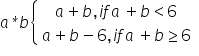Show that zero is the identity for this operation and each element a ≠ 0 of the set is invertible with 6 − a being the inverse of a.

Let X = {0, 1, 2, 3, 4, 5}.

The operation * on X is defined as ðÂ‘Å½ ∗ ðÂ‘Â =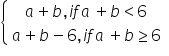An element e ∈ X is the identity element for the operation *, if

a ∗e = a = e ∗ a for all a ∈ X

For a ∈ X, we have

a ∗ 0 = a + 0 = a               [a ∈ X ⇒ a + 0 < 6]

0 ∗ a = 0 + a = a               [a ∈ X ⇒ 0 + a < 6]

∴ a ∗ 0 = a = 0 ∗ a for all a ∈ X

Thus, 0 is the identity element for the given operation *.

An element a ∈ X is invertible if there exists b ∈ X such that a * b = 0 = b * a.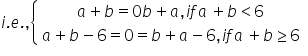⇒ a = −b or b = 6 − a

But, X = {0, 1, 2, 3, 4, 5} and a, b ∈ X. Then, a ≠ −b.

∴ b = 6 − a is the inverse of a for all a ∈ X.

Hence, the inverse of an element a ∈X, a ≠ 0 is 6 − a i.e., a −1 = 6 − a.

Question 15: Let A = {−1, 0, 1, 2}, B = {−4, −2, 0, 2} and f, g: A → B be functions defined by f(x) = x2 − x, x ∈ A and (ðÂ‘¥) = 2 |ðÂ‘¥ – (1/ 2) | − 1, ðÂ‘¥ ∈ A. Are f and g equal? Justify your answer. (Hint: One may note that two function f: A → B and g: A → B such that f(a) = g(a) ∀ a ∈ A, are called equal functions).

It is given that A = {−1, 0, 1, 2}, B = {−4, −2, 0, 2}.

Also, it is given that f, g: A → B are defined by

f(x) = x2 − x, x A and g(x) = 2 |x – (1/2) | − 1, x ∈ A

It is observed that

(−1) = (−1)2 − (−1) = 1 + 1 = 2

and g(−1) = 2 |(−1) – (1/2) | − 1 = 2 ( 3/2 ) − 1 = 3 − 1 = 2

⇒ (−1) = g(−1)

(0) = (0)2 − (0) = 0

and g(0) = 2 |0 – (1/2) | − 1 = 2 (1/2) − 1 = 1 − 1 = 0

⇒ (0) = g(0)

(1) = (1)2 − (1) = 1 − 1 = 0

and g(1) = 2 |(1) – (1/2) | − 1 = 2 (1/2) − 1 = 1 − 1 = 0

⇒ (1) = g(1)

(2) = (2)2 − (2) = 4 − 2 = 2

And (2) = 2 |(2) − 1 2 | − 1 = 2 (3/2) − 1 = 3 − 1 = 2

⇒ (2) = g(2) ∴ g(a) = g(a) for all a ∈ A

Hence, the functions f and g are equal.

Question 16: Let A = {1, 2, 3}. Then number of relations containing (1, 2) and (1, 3) which are reflexive and symmetric but not transitive is

(A) 1

(B) 2

(C) 3

(D) 4

The given set is A = {1, 2, 3}.

The smallest relation containing (1, 2) and (1, 3) which is reflexive and symmetric, but not transitive is given by:

R = {(1, 1), (2, 2), (3, 3), (1, 2), (1, 3), (2, 1), (3, 1)}

This is because relation R is reflexive as (1, 1), (2, 2), (3, 3) ∈ R.

Relation R is symmetric since (1, 2), (2, 1) ∈ R and (1, 3), (3, 1) ∈ R.

But relation R is not transitive as (3, 1), (1, 2) ∈ R, but (3, 2) ∉ R.

Now, if we add any two pairs (3, 2) and (2, 3) (or both) to relation R, then relation R will become transitive.

Hence, the total number of desired relations is one.

Question 17: Let A = {1, 2, 3}. Then number of equivalence relations containing (1, 2) is

(A) 1

(B) 2

(C) 3

(D) 4

It is given that A = {1, 2, 3}.

The smallest equivalence relation containing (1, 2) is given by,

R1 = {(1, 1), (2, 2), (3, 3), (1, 2), (2, 1)}

Now, we are left with only four pairs i.e., (2, 3), (3, 2), (1, 3), and (3, 1).

If we odd any one pair [say (2, 3)] to R1, then for symmetry we must add (3, 2).

Also, for transitivity we are required to add (1, 3) and (3, 1).

Hence, the only equivalence relation (bigger than R1) is the universal relation. This shows that the total number of equivalence relations containing (1, 2) is two.

Question 18: Let f: R → R be the Signum Function defined as (x) =and g: R → R be the Greatest Integer Function given by g(x) = [x], where [x] is greatest integer less than or equal to x. Then does fog and gof coincide in (0, 1]?

It is given that, f: R → R is defined as (ðÂ‘¥) =Also, g: R → R is defined as g(x) = [x], where [x] is the greatest integer less than

or equal to x.

Now, let x (0, 1].

Then, we have

[x] = 1 if x = 1 and [x] = 0 if 0 < x < 1.

fog(x) = f(g(x)) = f([x]) =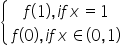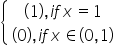go(x) =g(f(x)) = g(1)                  [ As  x > 0]

=  = 1

Thus, when x ∈ (0, 1), we have fog(x) = 0 and gof (x) = 1.

Hence, fog and gof do not coincide in (0, 1].

Question 19: Number of binary operations on the set {a, b} are

(A) 10

(B) 16

(C) 20

(D) 8

Answer 19: A binary operation * on {a, b} is a function from {a, b} × {a, b} → {a, b} i.e., * is a function from {(a, a), (a, b), (b, a), (b, b)} → {a, b}.

Hence, the total number of binary operations on the set {a, b} is 24 i.e., 16.